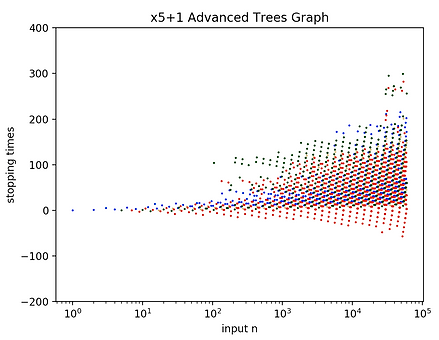top of page## Bonus Chapter: Divergents Trees

It was about this time that I began to consider the notion of divergent Collatz trees. A convergent tree, like the n=1 tree in x3+1 space, is a full connected system all of its own with many many branches up above and a single convergent loop at the bottom. If a convergent Collatz tree is a connected graph of Collatz hailstones which all share the same fate, the same convergent loop, could we not define an analogous concept of a connected graph of Collatz hailstones which all share the same divergent fate, the same diverging path to infinity?

Consider, as an example, the x5+1 world. The first (apparently) divergent hailstone we encounter is n=7. It sails up above our divergence cutoff of 10^100 and lands on a gigantic integer 100 digits long which ends in 6354166. The next two divergent hailstones are n=9 and n=11. Both sail up above the divergence cutoff and land on the same gigantic integer ending in 6354166. This merging of their paths happens as follows:

7 >> 36 >> 18 >> 9 >> 46 >> 23 >> 116 >> 58 >> 29 >> ...

9 >> 46 >> 23 >> 116 >> 58 >> 29 >> ...

11 >> 56 >> 28 >> 14 >> 7 >> 36 >> 18 >> 9 >> 46 >> 23 >> 116 >> 58 >> 29 >> ...

and forever thereafter they follow the same path.

But not all divergent hailstones arrive to that same high up value. The first one which seemingly takes its own path to infinity is n=21. It crosses the divergence cutoff of 10^100 and lands on its own gigantic integer 100 digits long ending in 7523416. No matter how much further I've ever looked these two paths to infinity do not ever seem to merge. (Trying to prove that, however, is fascinatingly impossible and is probably at least as difficult as the original Collatz Conjecture.)

Thus we may, with a little imagination, classify divergent hailstones by which "divergent tree" they are in, distinguished by which path they take to infinity. On a practical note we can distinguish them by which value they hit as they crossed the divergence cutoff.

Studying these divergent trees for a while, I began to wish to see what they looked like. My goal became to visually graph these divergent trees onto the Stopping Times graph, so we could actually see them standing next to the convergent trees and compare. However, before that would be possible first we would need to define a notion of a stopping time for a divergent hailstone. We could let it simply be the number of steps it took to cross the divergence cutoff, but then a small hailstone like n=7 would get an enormous stopping time. I invented the following idea instead...

Let's define the "trunk" of a divergent Collatz tree to be the smallest n value that connects to the tree. In the example above, the trunk would be n=7. It would be nice if the trunk of the tree had a stopping time of 0, so let us do the following: Let's define the stopping time for a divergent hailstone to be the number of steps it took for it to reach the divergence cutoff minus the number of steps it took for the trunk of that same tree to reach the divergence cutoff. Thus in the above example 7 would have a stopping time of 0, 11 would have a stopping time of 4, and 9 would have a stopping time of -3. Let's now show the graph of the x5+1 world both with and without the divergent tree at n=7.

It's really interesting to finally actually see one of the divergent trees nestled in next to the convergent ones! ... If we take the time now to add in all of the divergent trees, not just the one at n=7, we can finally see what the x5+1 Collatz world really looks like. The wild untamed divergent trees overwhelmingly dominate the forest of x5+1 spacebottom of page